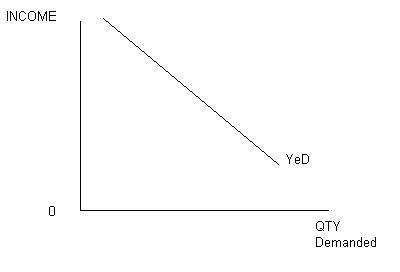# Elasticity of Demand

Elasticity is the ratio of the percent change in one variable to the percent change in another variable. It is a tool for measuring the responsiveness of a function to changes in parameters in a unit-less way.

ELASTICITY OF DEMAND

If price rises by 10% - what happens to demand? We know demand will fall

By more than 10%?

By less than 10%?

Elasticity measures the extent to which demand will change

Elasticity is the ratio of the percent change in one variable to the percent change in another variable. It is a tool for measuring the responsiveness of a function to changes in parameters in a unit-less way. Frequently used elasticities include price elasticity of demand, price elasticity of supply, income elasticity of demand, elasticity of substitution between factors of production and elasticity of intertemporal substitution

1. PRICE ELASTICITY OF DEMAND

Price elasticity of demand measures the percentage change in quantity demanded caused by a percent change in price. As such, it measures the extent of movement along the demand curve. This elasticity is almost always negative and is usually expressed in terms of absolute value. If the elasticity is greater than 1 demand is said to be elastic; between zero and one demand is inelastic and if it equals one, demand is unit-elastic.

E=  Proportionate change in qty demanded of good x / Proportionate change in price of good x

Calculating the Percentage Change in Quantity Demanded

The formula used to calculate the percentage change in quantity demanded is:

[QDemand(NEW) - QDemand(OLD)] / QDemand(OLD)

Calculating the Percentage Change in Price

Similar to before, the formula used to calculate the percentage change in price is:

[Price(NEW) - Price(OLD)] / Price(OLD)

PEoD = (% Change in Quantity Demanded)/(% Change in Price)

ELASTIC DEMAND - a change in price, results in a greater than proportional change in the quantity demanded ED>1.

INELASTIC DEMAND - a change in price results in a less than proportional change ED<1.

UNITARY DEMAND - a change in price results in n equal proportional change ED=1.

PERFECTLY ELASTIC DEMAND - demand changes even when price remains unchanged. ED=PERFECTLY INELASTIC DEMAND - change in price does not result in any change.

ED=0

2. Income elasticity of demand

Income elasticity of demand measures the percentage change in demand caused by a percent change in income. A change in income causes the demand curve to shift reflecting the change in demand. YED is a measurement of how far the curve shifts horizontally along the X-axis. Income elasticity can be used to classify goods as normal or inferior. With a normal good demand varies in the same direction as income. With an inferior good demand and income move in opposite directions.(Represented by 'YED')

The Income Elasticity of Demand: responsiveness of demand to changes in incomes

A positive sign denotes a normal good

A negative sign denotes an inferior good

MEASURING THE INCOME ELASTICITY

Income elasticity of demand (Yed) measures the relationship between a change in quantity demanded and a change in real income

Yed =             % change in demand

% change in income

TYPES OF INCOME ELASTICITY

POSITIVE INCOME ELASTICITY

A rise in income will cause a rise in demand

A fall in income will cause a fall in demand

NEGATIVE INCOME ELASTICITY

An increase in income will result in a decrease in demand.

A decrease in income will result in a rise in demand.

ALSO known as INFERIOR GOODS

Diagram of negative income elasticityZERO INCOME ELASTICITIES

This occurs when a change in income has NO effect on the demand for goods.

A rise of 5% income in a rich country will leave the Demand for toothpaste unchanged

3. Cross Elasticity:

The responsiveness of demand of one good to changes in the price of a related good – either a substitute or a complement

In economics, the cross elasticity of demand or cross-price elasticity of demand measures the responsiveness of the demand for a good to a change in the price of another good.

It is measured as the percentage change in demand for the first good that occurs in response to a percentage change in price of the second good. For example, if, in response to a 10% increase in the price of fuel, the demand of new cars that are fuel inefficient decreased by 20%, the cross elasticity of demand would be −20%/10% = −2.

Study Material, Lecturing Notes, Assignment, Reference, Wiki description explanation, brief detail
Engineering Economics and Financial Accounting : Demand and Supply Analysis : Elasticity of Demand |

Related Topics